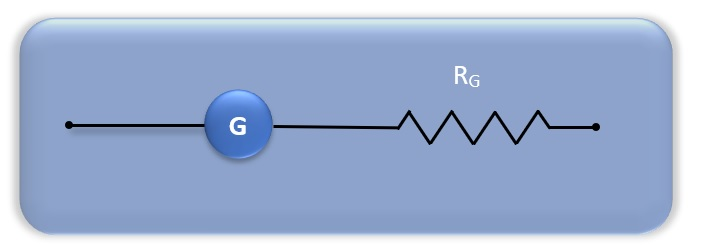Conversion of Galvanometer to Voltmeter

# Our Objective

To convert the given galvanometer (of known resistance and figure of merit) into a voltmeter of desired range (say 0 to 3 V) and to verify the same.

# The Theory

## Galvanometer

A galvanometer is a sensitive device which can detect the presence of very small current in a circuit of the order of 100 mA. Its working is based on the principle that a coil placed in a uniform magnetic field experiences a torque when an electric current is set up in it. This deflection of the coil is determined by a pointer attached to it, moving on a scale. The pointer lies in its neutral position in the middle of the scale when no current is flowing through it. Depending on the direction of the current, the pointer deflection is either to the right or the left.

## Voltmeter

A voltmeter is a device used to measure the potential difference between two points in an electric circuit. It is always connected in parallel to the circuit so that the voltage drops across it is same as the voltage drops across the required section of the circuit.

## Converting galvanometer to a voltmeter

A galvanometer has low resistance and hence large current flows through it. Due to this, the current and potential difference across a conductor decrease. Hence it cannot be used to measure the potential difference across a conductor. But we can convert a galvanometer into a voltmeter by connecting a large resistance in series to the galvanometer. This will ensure that the galvanometer draws very small current from the circuit. Hence, voltage measurement will not have much effect on the original circuit setup.Fig: Converted galvanometer

To measure the potential difference across a section of the circuit we must connect the voltmeter or the converted galvanometer in parallel with the required section of the circuit. If a galvanometer shows a full scale deflection for a maximum current IgIg then,

Ig=Nk

Ig= The current required to produce full scale deflection in the galvanometer

N = total number of divisions N on either side of the zero of galvanometer

k = figure of merit of galvanometer

The potential difference across the galvanometer having resistance G is Ig G. On connecting a series resistance R with galvanometer, the potential difference V across the section becomes

V = (R + G) I

Since IgIg is the current required to produce full scale deflection in the galvanometer, if the converted galvanometer is desired to have a range V volt, then the resistance to be joined in series with galvanometer,

R=VIg−GR=VIg−G

That is, the desired range of V volts in voltmeter will be shown by the galvanometer having a total of N divisions.

N divisions of converted galvanometer = V volt.

The least count of the converted galvanometer, L.C =V/N

Hence, we can convert the reading of galvanometer to volts using the relation

VG = n x L.C

VG = Potential difference in volts

n = No. of divisions in galvanometer

L.C = Least count of the converted voltmeter

A standard voltmeter connected in parallel to the converted galvanometer can be used to verify the error in value of potential difference measured by the converted galvanometer.

# Learning Outcomes

The student learns the following concepts:

• Galvanometer
• Voltmeter
• How a galvanometer can be converted into a voltmeter.